# numpy.random.RandomState.poisson¶

RandomState.poisson(lam=1.0, size=None)

Draw samples from a Poisson distribution.

The Poisson distribution is the limit of the Binomial distribution for large N.

Parameters: lam : float or sequence of float Expectation of interval, should be >= 0. A sequence of expectation intervals must be broadcastable over the requested size. size : int or tuple of ints, optional Output shape. If the given shape is, e.g., (m, n, k), then m * n * k samples are drawn. Default is None, in which case a single value is returned.

Notes

The Poisson distribution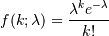For events with an expected separation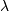the Poisson distribution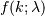describes the probability of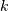events occurring within the observed interval.

Because the output is limited to the range of the C long type, a ValueError is raised when lam is within 10 sigma of the maximum representable value.

References

 [R163] Weisstein, Eric W. “Poisson Distribution.” From MathWorld–A Wolfram Web Resource. http://mathworld.wolfram.com/PoissonDistribution.html
 [R164] Wikipedia, “Poisson distribution”, http://en.wikipedia.org/wiki/Poisson_distribution

Examples

Draw samples from the distribution:

>>> import numpy as np
>>> s = np.random.poisson(5, 10000)


Display histogram of the sample:

>>> import matplotlib.pyplot as plt
>>> count, bins, ignored = plt.hist(s, 14, normed=True)
>>> plt.show()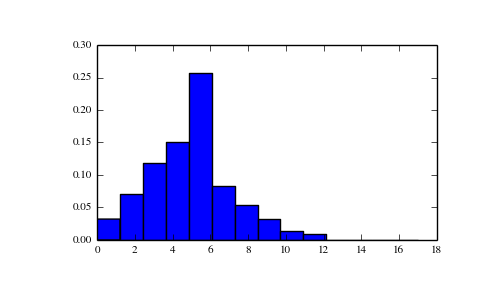Draw each 100 values for lambda 100 and 500:

>>> s = np.random.poisson(lam=(100., 500.), size=(100, 2))


()

#### Previous topic

numpy.random.RandomState.permutation

#### Next topic

numpy.random.RandomState.power